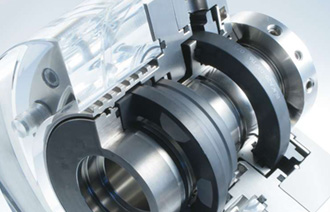# Energy saving principle analysis of variable frequency pump

Update: 25-07-2020

Abst:

[frequency conversion] has become the synonym of energy […]

[frequency conversion] has become the synonym of energy saving in this era. Is frequency conversion electric appliance really energy-saving? What is the energy-saving principle of frequency conversion water pump? Let's see the following analysis:
According to fluid mechanics, the actual power P (power) = q (flow) ≛ H (pressure), the flow rate q is directly proportional to the first power of speed n, the pressure h is proportional to the square of speed n, and the power P is proportional to the cube of speed n. if the efficiency of the water pump is constant, when the regulated flow rate is required to decrease, the speed n can decrease proportionally, and the output power P of the shaft will decrease in cubic relationship.

To sum up: that is, the power consumption of the pump motor and the speed of the approximate square ratio.
For example: a pump motor power is 55kW, when the speed drops to 4 / 5 of the original speed, its power consumption is 28.16kw, saving 48.8%; when the speed drops to 1 / 2 of the original speed, the power consumption is 6.875kw, saving 87.5%. The power factor compensation of frequency conversion water pump can save energy Reactive power not only increases line loss and equipment heating, but also reduces the power factor, which leads to the reduction of active power in the power grid. A large amount of reactive power is consumed in the line, and the equipment is inefficient and wasted seriously. According to the formula P = s ╳ cos Ф, q = s ≛ sin Ф, where s-apparent power, p-active power, q-reactive power, cos Ф - power factor, it can be seen that the larger cos Ф, there are The greater the power P, the power factor of the ordinary water pump motor is between 0.6 and 0.7. After using the frequency conversion speed regulating device, due to the function of the filter capacitor inside the frequency converter, cos Ф≈ 1, thus reducing the reactive power loss and increasing the active power of the grid.

Ningbo Sanxin Pump Co., Ltd.1+